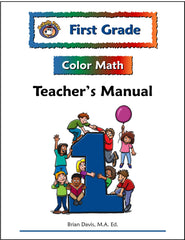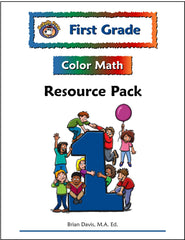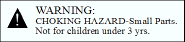# First Grade Color Math Curriculum with Manipulative Kit

9781592691463

The McRuffy First Grade Color Math Curriculum with Manipulative Kit Package includes:

• Teacher's Manual
• Workbook
• Resource Pack
• Manipulative Kit
First Grade Color Math Teacher's Manual and Workbook Sample

The objectives of the  First Grade Color Math Curriculum  are chosen to match state and national math standards. Skills are not just introduced but revisited throughout the year to allow for the child’s growth in understanding. The curriculum places a special emphasis on addition and subtraction skills. Along the way a great variety of concepts emphasizing geometry, number handwriting, listening skills, place value, and more round out the first grade program.

Concepts include: Number Recognition to 1000,  Skip counting by 2, 5, 10, and 100, Recognizing and making patterns, Recognizing shapes, Making geometric designs using pattern blocks, inch cubes, and tangrams, Visual discrimination skills using geoboard patterns, Comparing numbers and groups using arrows to represent < and >, Reading and writing times to the half-hour, Measuring using inches and centimeters, Unit conversions for length, Liquid measurement, Story problems, Game playing (counting skills, following directions, etc.), Place value (hundreds, tens and ones), Number sentences, Using symbols to represent numbers, Counting coins (pennies, nickels, dimes, quarters), Addition up to three-digits, Addition with regrouping, Addition with three addends, Subtraction up to three-digits, Commutative property of addition and multiplication (1+2=2+1), Fractions (half), Attributes, Odd and Even, Number words (zero to twenty), Symmetry,  Multiplication by 2, Thermometer reading, Estimating time and temperature, Rounding numbers to the nearest ten, Making and reading charts, tables, and graphs.The First Grade Color Math Teacher’s Manual  contains easy-to-use daily lesson plans. Lesson objectives are clearly stated at the beginning of the lesson so you’ll know exactly what you’re teaching. The Materials section of the lesson plan states the materials and manipulative used in the lesson so you’ll be fully prepared to teach. Most lessons require very little advanced preparation.The McRuffy Press First Grade Color Math Workbook gives students the opportunity to practice and demonstrate skills. Most pages contain two activities. Activities include place value, measuring, graphs, number writing, coin counting, skip counting, number lines, inequalities, reading thermometers, geometric puzzles, patterns, clock reading, addition, subtraction, and fractions of objects.The Resource Pack contains an extensive collection of unbound materials. It includes additional activity sheets, unit tests, timed tests, games, laminated geoboards, posters, learning mats, number lines, attribute shapes, and precut cards. The Resource Pack expands the opportunities to learn and support instruction.

The First Grade Color Math Sample includes pages from the Teacher’s Manual and corresponding workbook page.First Grade Color Math Manipulative Kit

Our first grade math lessons use popular math manipulatives. The manipulative kit has everything you need to fully utilize the First Grade Color Math Curriculum. Manipulatives make the concepts come alive. The kit includes:

• 25 Animal Counters
• Base Ten Set: 1 Base Ten Flat, 20 Base Ten Rods, 100 Base Ten Centimeter Cubes,
• 20 10-color Centimeter Cubes
• 12 One Inch Cubes
• Geoboard with rubber bands
• 250 Counting Chips
• 50 Pattern Blocks
• 7 Piece Tangram set
• Clock Dial
• Game Playing Piece Set